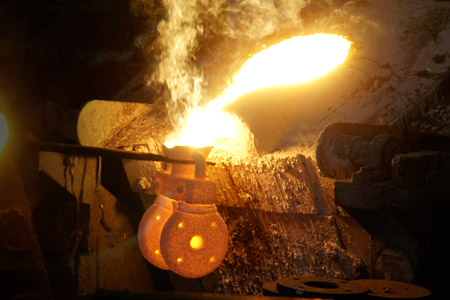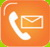About Us | Metal Casting | Casting Workshop | Machining Workshop | Quality Inspection | Contact Us

The Importance of Cost Estimation in Investment Casting"Investment casting made in china" is booming at a rapid pace and looking at the present scenario one concept that has gained its popularity in past couple of years is "Investment Casting Cost Estimation". These days the competition has grown at the phenomenon rate and in order to survive and compete at a global platform, investment casting industry has to meet ever increasing customers' expectations in terms of quality standards and lower pricing. Investment casting process planning generally consists of proper choice of suitable casting process and various investment casting materials. Now in order to have continuous cost reduction in casting process, it is essential to build up an easy-to-use casting cost estimation methodology. It is also important to note that any comprehensive investment casting cost estimation methodology must have ability to identify the most important parameters in casting cost. The most important attributes of investment casting are cost estimation of material and tooling process. Material Cost Estimation In investment casting material cost estimation the two vital factors that play the major role are cost estimation of in-direct and direct materials. Direct Material Cost: Now in case of direct material cost, the cost estimation is made on materials like cast metal or alloy that appears in any product constitutes. However, in case of direct material cost, the metals or alloys that are used can be determined from weight of investment casting. Direct material cost can be measured as: C = c unit_metal x; wcast × fm ×fp ×ff Where, Cunit_metal = Unit metal cost Wcast = Casting weight = pc × Vcast pc= Casting metal density Vcast = Casting volume fm, fp, ff = Factors for metal loss in melting, pouring and fettling respectively In-direct Material Cost: Depending upon the casting process in-direct materials consist of moulding sand, dispensable cores and feeding aids. Moreover the most essential point that one need to consider in case of in-direct material cost is the price of moulding sand. The price of moulding sand actually relies upon on the type and composition of sand, and size of mould. The cost of core ore sand also depends on the kind of sand and quantity of cores. In addition to this the cost modifiers are need to understood very effectively for mould rejection or core rejection. Indirect material cost can be calculated as: x frm/n mould sand= Cms xWms frm /nc Cost core sand = Cs Wcs fcc frc Where, nc= Number of casting (cavities) per mould Cms= Cost per unit weight of mould sand (1NR/Kg) Ccs = Cost per unit weight of core sand (1NR/Kg) frm = Mould rejection factor f cm = Mould sand recycling factor f cc = Core sand recycling factor f rc = Core rejection factor Wms= Weight of mould sand Wcs= Weight of core sand Now the point to be noted is in case of indirect material cost sand recycling factor plays a very significant function. It helps in indicating the actual quantity of new sand required for each mould or core. The rejection factor is considered to measure the account breakages or damages. Moreover, the mould sand cost is finally divided by the number of casting cavities to find the price of mould sand cost per casting. Tooling Cost Estimation Tooling is the most important aspect in casting and thus its cost estimation can't be simply ignored. It consists of outline and core box for sand casting and metal mould for die casting in addition to investment casting. Their cost is determined by the material and manufacturing of the tooling and the material is selected depending upon the tool life required, which is further influenced by the order quantity. Certainly the tool manufacturing cost is determined by its geometric complexity and the complexity can be calculated as: X=A0+AaCa+AcCc where A0=Regression coefficients Ca=Area complexity factor =I-(surface area of cube of equal volume/surface area of solid) =I-(6 V2/3/A) V=Volume of part A=Surface area of part Cc=Core complexity factor Nc=number of cores However, the important point to note in this case is the area complexity factor is normally calculated by evaluating the surface area of a part with respect to the surface area of a cube of equal volume. This is based on the assumption that a cube is the simplest shape and has the least surface area for a given volume. Today estimating the cost of investment casting has become one of the major factors for the growth of any metal casting industry. "How much does an investment casting cost in China?" The successful completion of any project largely depends on the proper and precise cost estimation of investment casting. As a project is proposed and then developed, an effective forecasting the cost of future projects is vital to the survival of any business or organization. From an owner's perspective the cost estimate actually help in determining the project scope. So if you are one of the players in this investment casting industry, make sure to have investment casting cost estimation for successful project completion.Liaoning Borui Machinery Co., Ltd. All rights reserved. Add: No.1-4-6, Jianshe Road, Qianyang, Donggang City, Dandong, Liaoning, China                    Cast Iron Foundry | Sitemap